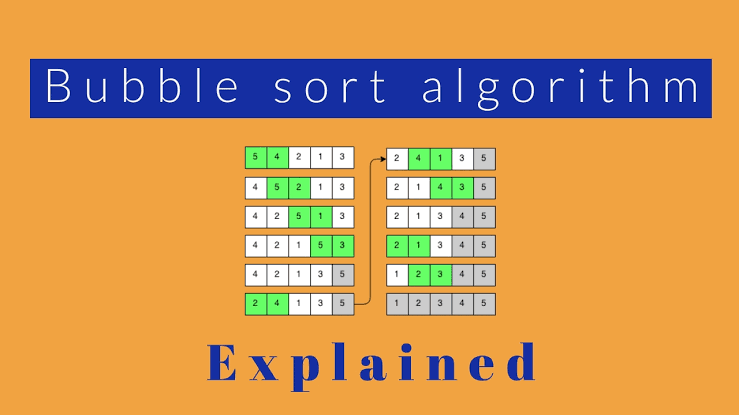To advertise with us contact on Whatsapp: +923041280395 For guest post email at: [email protected]

# Bubble Sort Implementation## Overview

There are limited ways to search a list that is unsorted. It is often more efficient to sort a list and then search it. One of the most basic sorting algorithms is called bubble sort. This algorithm gets its name from the way values eventually “bubble” up to their proper position in the sorted array.

This basic approach to sorting narrows the scope of our problem to focusing on ordering just two elements at a time, instead of an entire array at a time. This approach is very straightforward, but possibly at the expense of making an inordinate number of swaps just to put one single element into position.

## Bubble Sort Implementation

Bubble sort works by comparing two adjacent numbers in a list, and swapping them if they are out of order. Looking at the example on the left, if we are given an array of the numbers 5, 1, 6, 2, 4, and 3 and we wanted to sort it using bubble sort our pseudocode for one single pass might look something like this:

``````for every element in the array
check if element to the right is smaller
if so swap the two elements
else move on to the next element in the list``````

When this is implemented on the example array, the program would start at 5 and compare it with 1. Since 1 is smaller than 5 it would swap them. It would then move on to compare 5 and 6 since those are in the correct order, we just move on to the next element. Next 6 and 2 are compared, and so on.

Finally, after doing this for all the elements in the array, we are left with the array 1, 5, 2, 4, 3, and 6. It’s not completely sorted, but notice that after the first pass through, the 6 is already in its correct location. After n pass-throughs, the last n elements are in their correct position. This fact can be used to optimize this algorithm since it is not necessary to look at those correctly sorted elements. It is this effect of the larger elements “bubbling” to the right side that gives this algorithm its name!

## Sorted Arrays

If bubble sort was implemented only as above, we would only go through one passthrough, but as the example shows, it is not guaranteed that the array will be sorted after one pass. So how many times should this algorithm be run? Well in the worst-case scenario, a reverse sorted list (6, 5, 4, 3, 2, 1), it might need to run 5 times. Indeed the same would hold true for n elements, the algorithm might need to run n-1 times. That seems wasteful though since it would only need to run that maximum number of times if the array is a “worst-case scenario” (more on that in the time complexity module).

How can you ensure you only run this algorithm the necessary amount of times, maybe saving a few steps? Well, if this algorithm is run and no swaps are made, it must be true that the array is sorted (think about it)! Maybe then it would make sense to amend our implementation to include a counter for the number of swaps made. If counter == 0, then the array is sorted, however, if counter > 0, then more pass-throughs are needed to sort the
array. Now we only decide at the end of every passthrough whether more pass-throughs are necessary!